# Chapter 25 Electric Potential Electric Potential Electromagnetism has

• Slides: 51Chapter 25 Electric Potential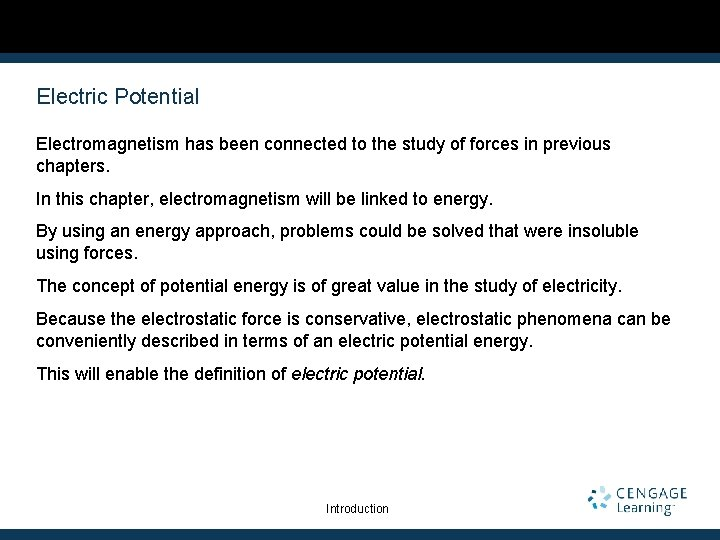Electric Potential Electromagnetism has been connected to the study of forces in previous chapters. In this chapter, electromagnetism will be linked to energy. By using an energy approach, problems could be solved that were insoluble using forces. The concept of potential energy is of great value in the study of electricity. Because the electrostatic force is conservative, electrostatic phenomena can be conveniently described in terms of an electric potential energy. This will enable the definition of electric potential. Introduction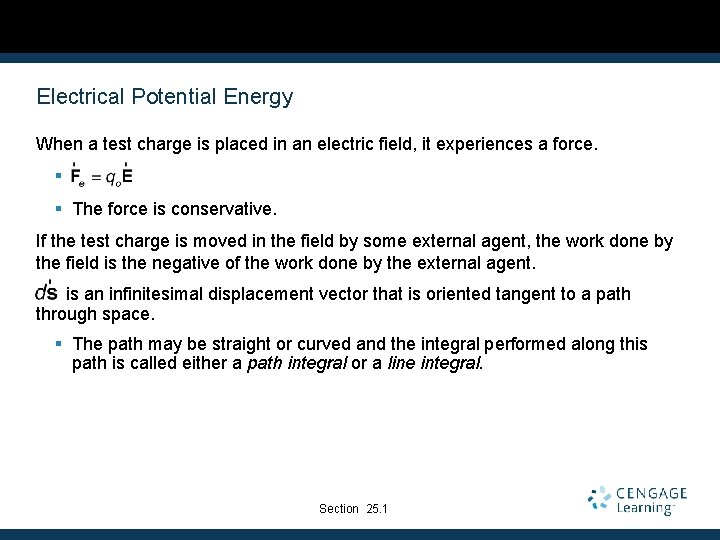Electrical Potential Energy When a test charge is placed in an electric field, it experiences a force. § § The force is conservative. If the test charge is moved in the field by some external agent, the work done by the field is the negative of the work done by the external agent. is an infinitesimal displacement vector that is oriented tangent to a path through space. § The path may be straight or curved and the integral performed along this path is called either a path integral or a line integral. Section 25. 1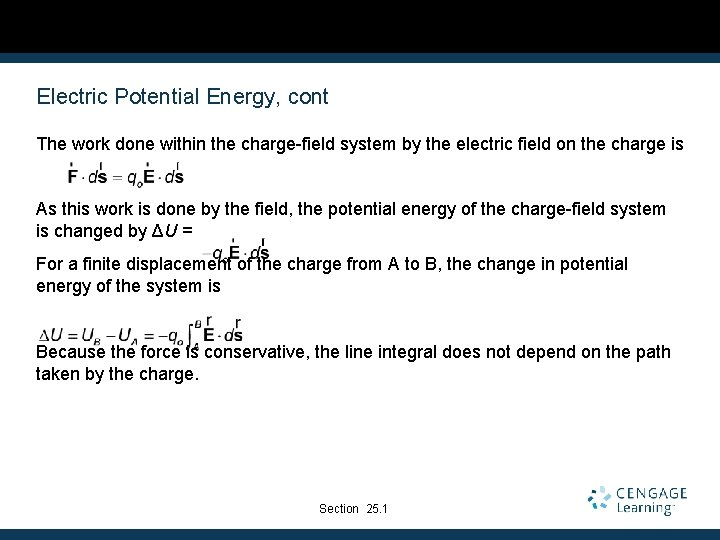Electric Potential Energy, cont The work done within the charge-field system by the electric field on the charge is As this work is done by the field, the potential energy of the charge-field system is changed by ΔU = For a finite displacement of the charge from A to B, the change in potential energy of the system is Because the force is conservative, the line integral does not depend on the path taken by the charge. Section 25. 1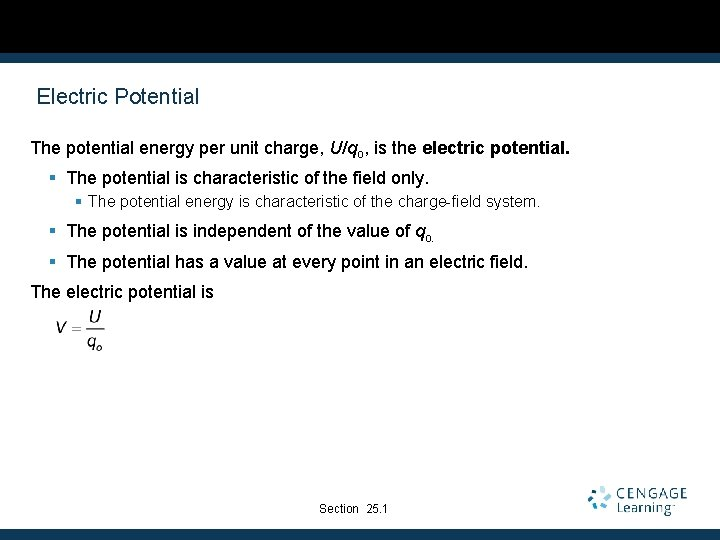Electric Potential The potential energy per unit charge, U/qo, is the electric potential. § The potential is characteristic of the field only. § The potential energy is characteristic of the charge-field system. § The potential is independent of the value of qo. § The potential has a value at every point in an electric field. The electric potential is Section 25. 1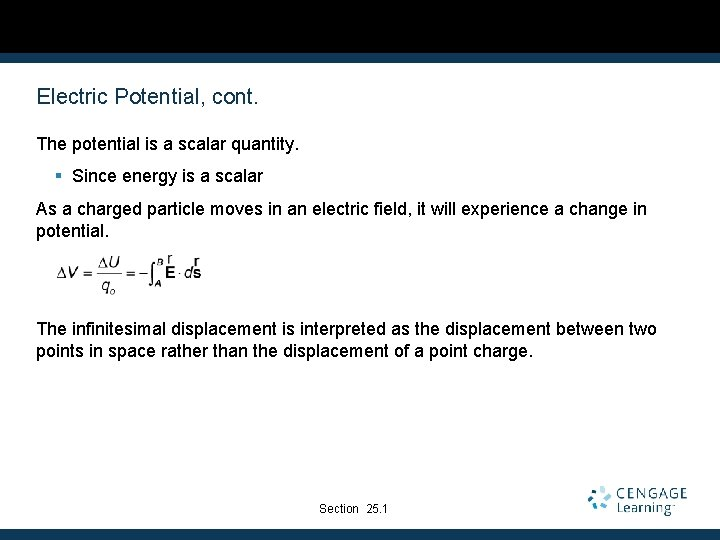Electric Potential, cont. The potential is a scalar quantity. § Since energy is a scalar As a charged particle moves in an electric field, it will experience a change in potential. The infinitesimal displacement is interpreted as the displacement between two points in space rather than the displacement of a point charge. Section 25. 1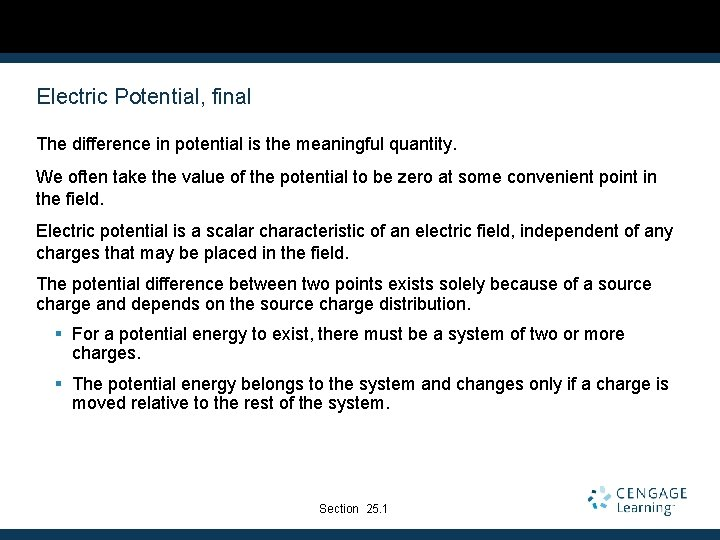Electric Potential, final The difference in potential is the meaningful quantity. We often take the value of the potential to be zero at some convenient point in the field. Electric potential is a scalar characteristic of an electric field, independent of any charges that may be placed in the field. The potential difference between two points exists solely because of a source charge and depends on the source charge distribution. § For a potential energy to exist, there must be a system of two or more charges. § The potential energy belongs to the system and changes only if a charge is moved relative to the rest of the system. Section 25. 1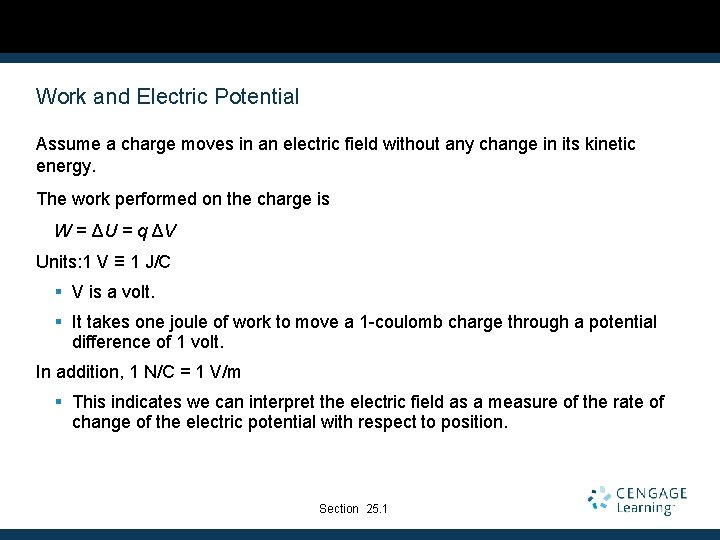Work and Electric Potential Assume a charge moves in an electric field without any change in its kinetic energy. The work performed on the charge is W = ΔU = q ΔV Units: 1 V ≡ 1 J/C § V is a volt. § It takes one joule of work to move a 1 -coulomb charge through a potential difference of 1 volt. In addition, 1 N/C = 1 V/m § This indicates we can interpret the electric field as a measure of the rate of change of the electric potential with respect to position. Section 25. 1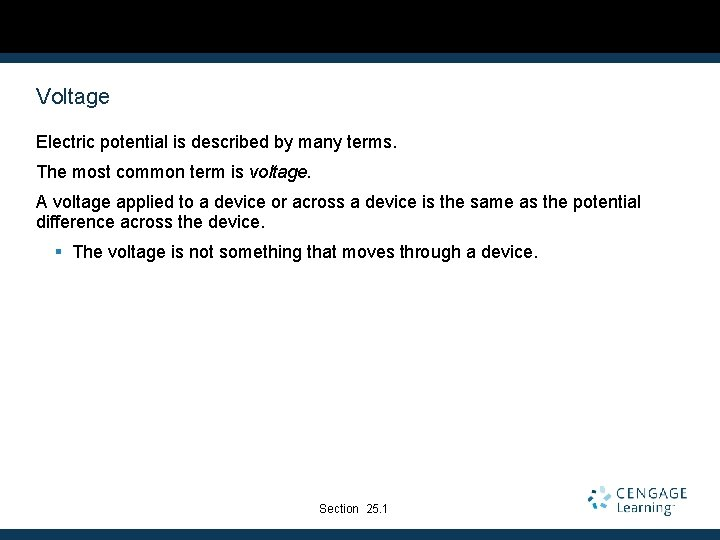Voltage Electric potential is described by many terms. The most common term is voltage. A voltage applied to a device or across a device is the same as the potential difference across the device. § The voltage is not something that moves through a device. Section 25. 1Electron-Volts Another unit of energy that is commonly used in atomic and nuclear physics is the electron-volt. One electron-volt is defined as the energy a charge-field system gains or loses when a charge of magnitude e (an electron or a proton) is moved through a potential difference of 1 volt. § 1 e. V = 1. 60 x 10 -19 J Section 25. 1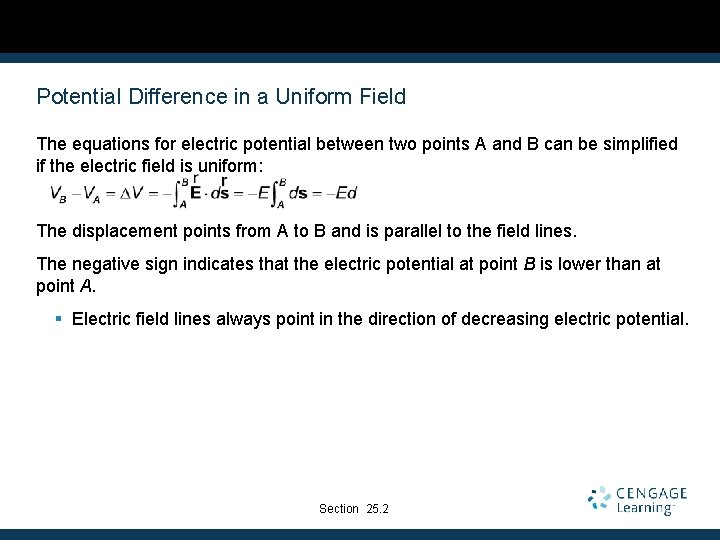Potential Difference in a Uniform Field The equations for electric potential between two points A and B can be simplified if the electric field is uniform: The displacement points from A to B and is parallel to the field lines. The negative sign indicates that the electric potential at point B is lower than at point A. § Electric field lines always point in the direction of decreasing electric potential. Section 25. 2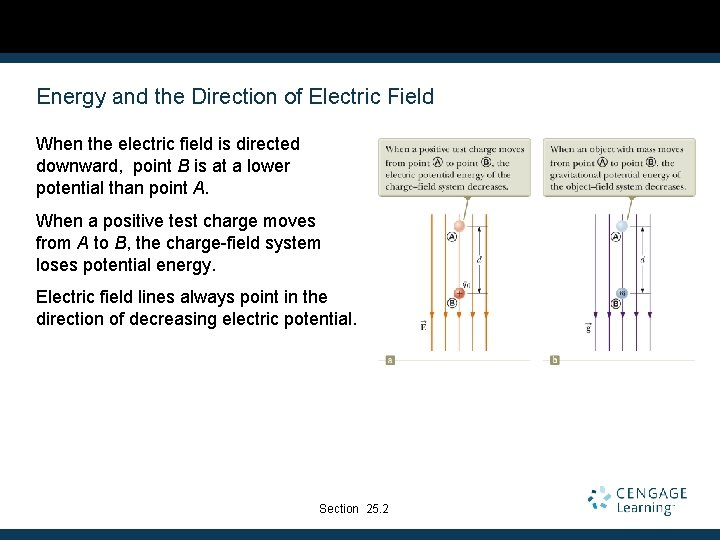Energy and the Direction of Electric Field When the electric field is directed downward, point B is at a lower potential than point A. When a positive test charge moves from A to B, the charge-field system loses potential energy. Electric field lines always point in the direction of decreasing electric potential. Section 25. 2More About Directions A system consisting of a positive charge and an electric field loses electric potential energy when the charge moves in the direction of the field. § An electric field does work on a positive charge when the charge moves in the direction of the electric field. The charged particle gains kinetic energy and the potential energy of the chargefield system decreases by an equal amount. § Another example of Conservation of Energy Section 25. 2Directions, cont. If qo is negative, then ΔU is positive. A system consisting of a negative charge and an electric field gains potential energy when the charge moves in the direction of the field. § In order for a negative charge to move in the direction of the field, an external agent must do positive work on the charge. Section 25. 2Equipotentials Point B is at a lower potential than point A. Points A and C are at the same potential. § All points in a plane perpendicular to a uniform electric field are at the same electric potential. The name equipotential surface is given to any surface consisting of a continuous distribution of points having the same electric potential. Section 25. 2Charged Particle in a Uniform Field, Example A positive charge is released from rest and moves in the direction of the electric field. The change in potential is negative. The change in potential energy is negative. The force and acceleration are in the direction of the field. Conservation of Energy can be used to find its speed. Section 25. 2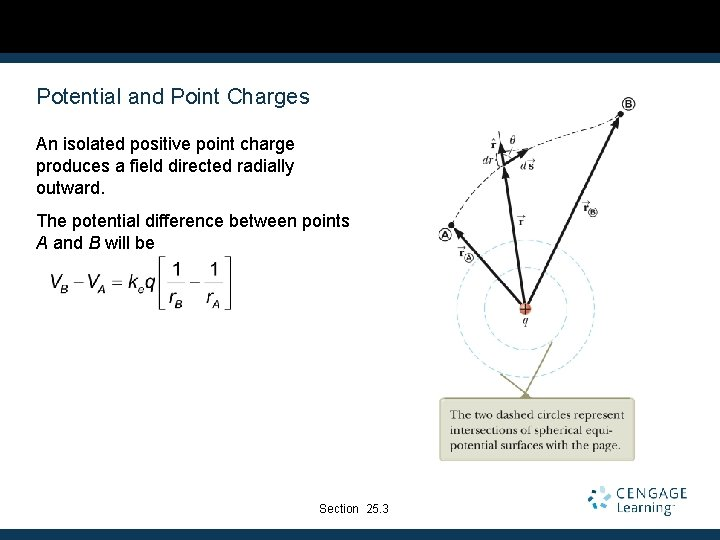Potential and Point Charges An isolated positive point charge produces a field directed radially outward. The potential difference between points A and B will be Section 25. 3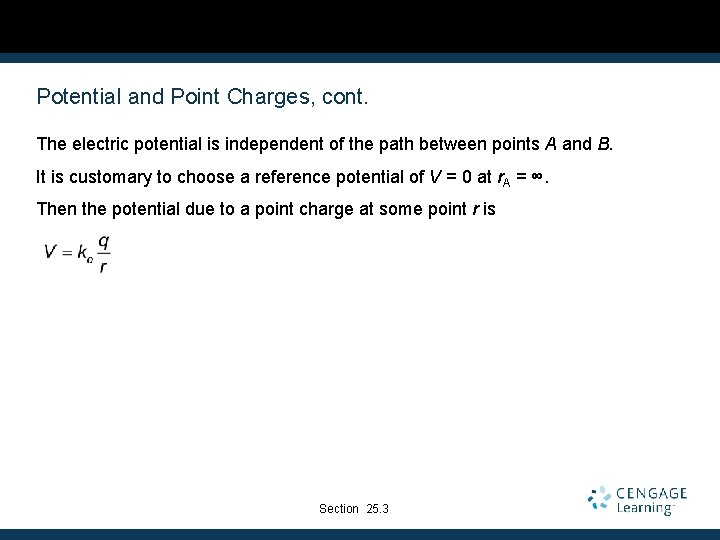Potential and Point Charges, cont. The electric potential is independent of the path between points A and B. It is customary to choose a reference potential of V = 0 at r. A = ∞. Then the potential due to a point charge at some point r is Section 25. 3Electric Potential of a Point Charge The electric potential in the plane around a single point charge is shown. The red line shows the 1/r nature of the potential. Section 25. 3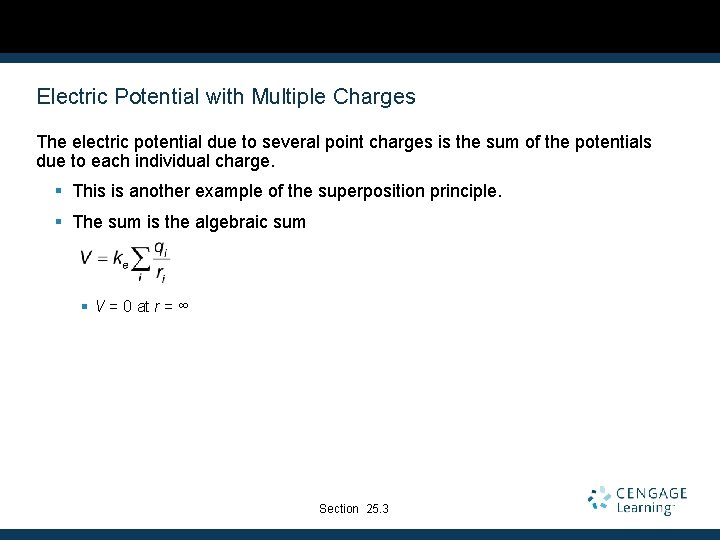Electric Potential with Multiple Charges The electric potential due to several point charges is the sum of the potentials due to each individual charge. § This is another example of the superposition principle. § The sum is the algebraic sum § V = 0 at r = ∞ Section 25. 3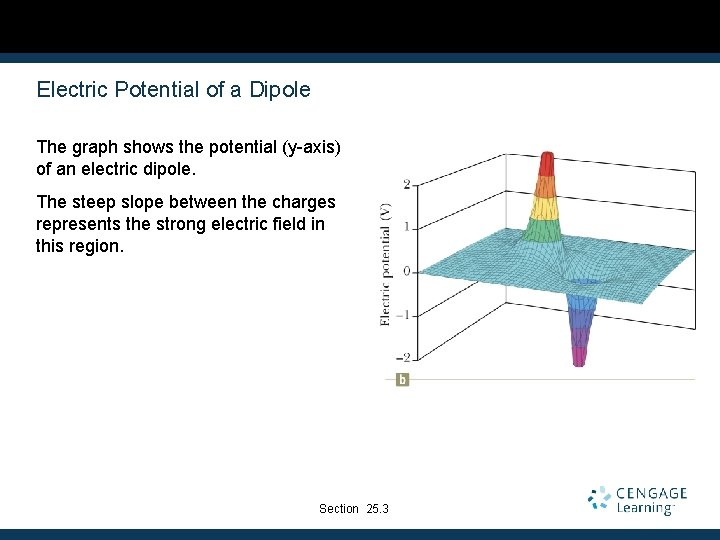Electric Potential of a Dipole The graph shows the potential (y-axis) of an electric dipole. The steep slope between the charges represents the strong electric field in this region. Section 25. 3Potential Energy of Multiple Charges The potential energy of the system is . If the two charges are the same sign, U is positive and work must be done to bring the charges together. If the two charges have opposite signs, U is negative and work is done to keep the charges apart. Section 25. 3U with Multiple Charges, final If there are more than two charges, then find U for each pair of charges and add them. For three charges: § The result is independent of the order of the charges. Section 25. 3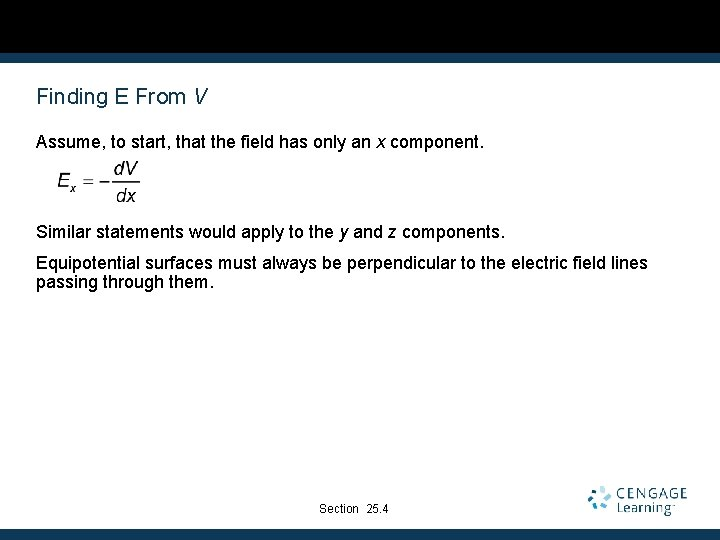Finding E From V Assume, to start, that the field has only an x component. Similar statements would apply to the y and z components. Equipotential surfaces must always be perpendicular to the electric field lines passing through them. Section 25. 4E and V for an Infinite Sheet of Charge The equipotential lines are the dashed blue lines. The electric field lines are the brown lines. The equipotential lines are everywhere perpendicular to the field lines. Section 25. 4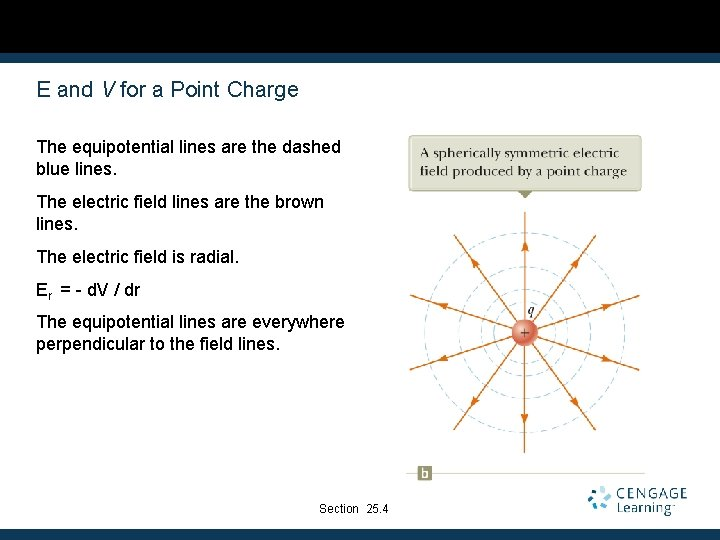E and V for a Point Charge The equipotential lines are the dashed blue lines. The electric field lines are the brown lines. The electric field is radial. Er = - d. V / dr The equipotential lines are everywhere perpendicular to the field lines. Section 25. 4E and V for a Dipole The equipotential lines are the dashed blue lines. The electric field lines are the brown lines. The equipotential lines are everywhere perpendicular to the field lines. Section 25. 4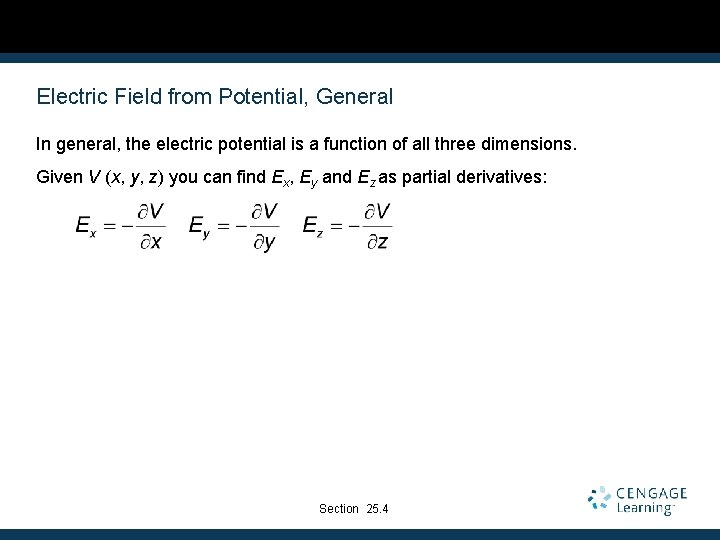Electric Field from Potential, General In general, the electric potential is a function of all three dimensions. Given V (x, y, z) you can find Ex, Ey and Ez as partial derivatives: Section 25. 4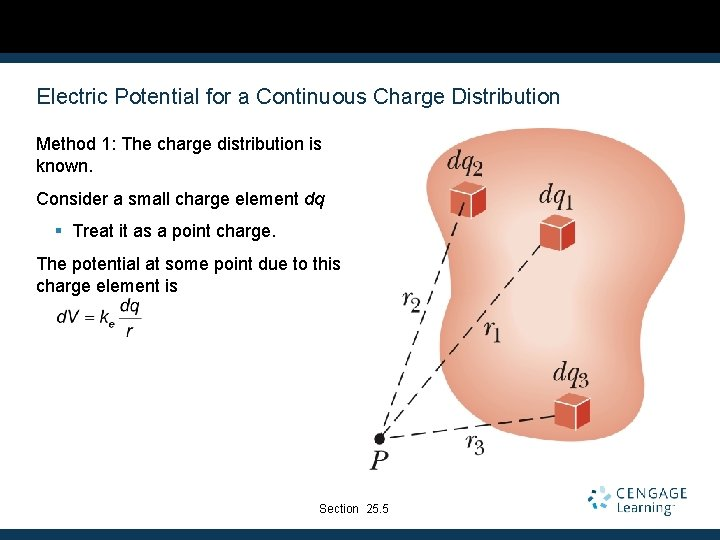Electric Potential for a Continuous Charge Distribution Method 1: The charge distribution is known. Consider a small charge element dq § Treat it as a point charge. The potential at some point due to this charge element is Section 25. 5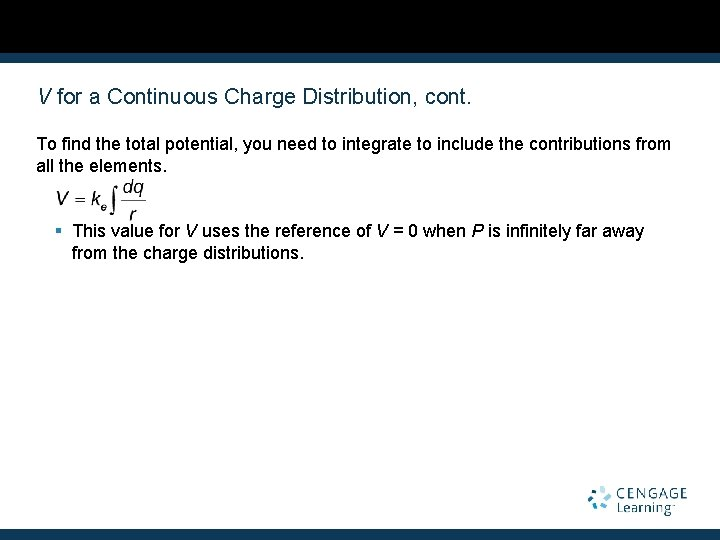V for a Continuous Charge Distribution, cont. To find the total potential, you need to integrate to include the contributions from all the elements. § This value for V uses the reference of V = 0 when P is infinitely far away from the charge distributions.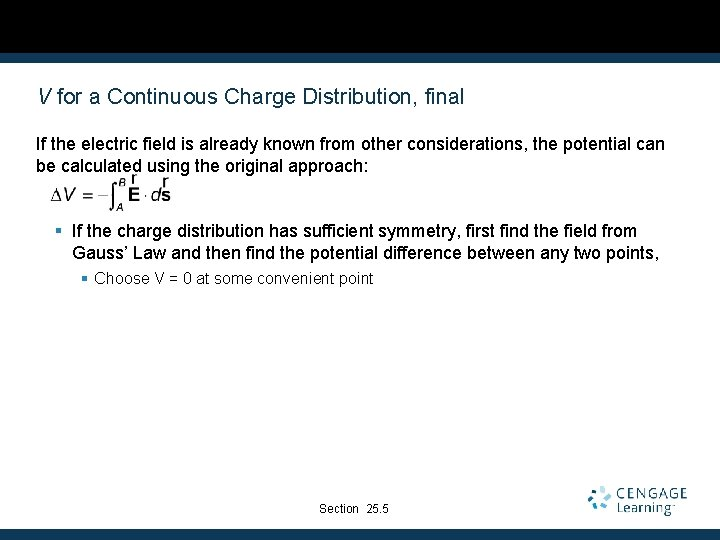V for a Continuous Charge Distribution, final If the electric field is already known from other considerations, the potential can be calculated using the original approach: § If the charge distribution has sufficient symmetry, first find the field from Gauss’ Law and then find the potential difference between any two points, § Choose V = 0 at some convenient point Section 25. 5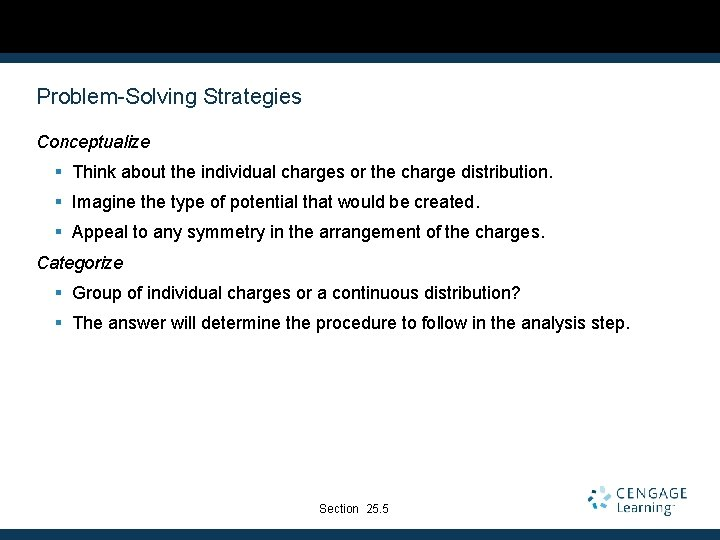Problem-Solving Strategies Conceptualize § Think about the individual charges or the charge distribution. § Imagine the type of potential that would be created. § Appeal to any symmetry in the arrangement of the charges. Categorize § Group of individual charges or a continuous distribution? § The answer will determine the procedure to follow in the analysis step. Section 25. 5Problem-Solving Strategies, 2 Analyze § General § Scalar quantity, so no components § Use algebraic sum in the superposition principle § Keep track of signs § Only changes in electric potential are significant § Define V = 0 at a point infinitely far away from the charges. § If the charge distribution extends to infinity, then choose some other arbitrary point as a reference point. Section 25. 5Problem-Solving Strategies, 3 Analyze, cont § If a group of individual charges is given § Use the superposition principle and the algebraic sum. § If a continuous charge distribution is given § Use integrals for evaluating the total potential at some point. § Each element of the charge distribution is treated as a point charge. § If the electric field is given § Start with the definition of the electric potential. § Find the field from Gauss’ Law (or some other process) if needed. Section 25. 5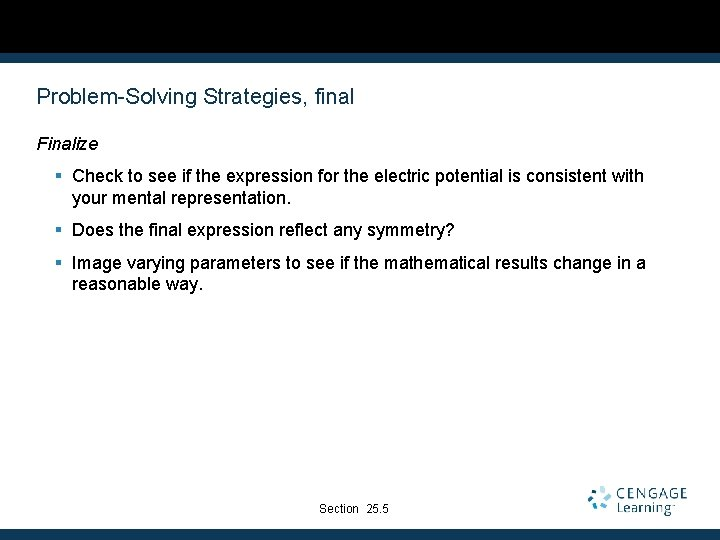Problem-Solving Strategies, final Finalize § Check to see if the expression for the electric potential is consistent with your mental representation. § Does the final expression reflect any symmetry? § Image varying parameters to see if the mathematical results change in a reasonable way. Section 25. 5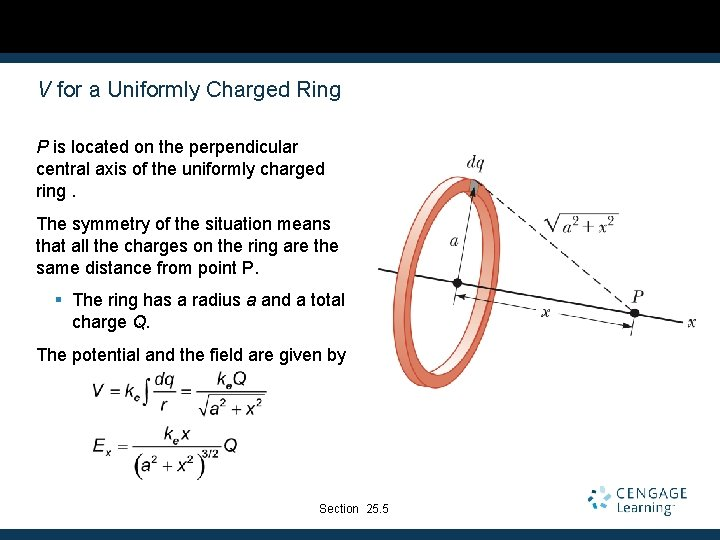V for a Uniformly Charged Ring P is located on the perpendicular central axis of the uniformly charged ring. The symmetry of the situation means that all the charges on the ring are the same distance from point P. § The ring has a radius a and a total charge Q. The potential and the field are given by Section 25. 5V for a Uniformly Charged Disk The ring has a radius R and surface charge density of σ. P is along the perpendicular central axis of the disk. P is on the cental axis of the disk, symmetry indicates that all points in a given ring are the same distance from P. The potential and the field are given by Section 25. 5V for a Finite Line of Charge A rod of line ℓ has a total charge of Q and a linear charge density of λ. § There is no symmetry to use, but the geometry is simple. Section 25. 5V Due to a Charged Conductor Consider two points on the surface of the charged conductor as shown. is always perpendicular to the displacement. Therefore, the potential difference between A and B is also zero. Section 25. 6V Due to a Charged Conductor, cont. V is constant everywhere on the surface of a charged conductor in equilibrium. § ΔV = 0 between any two points on the surface The surface of any charged conductor in electrostatic equilibrium is an equipotential surface. Every point on the surface of a charge conductor in equilibrium is at the same electric potential. Because the electric field is zero inside the conductor, we conclude that the electric potential is constant everywhere inside the conductor and equal to the value at the surface. Section 25. 6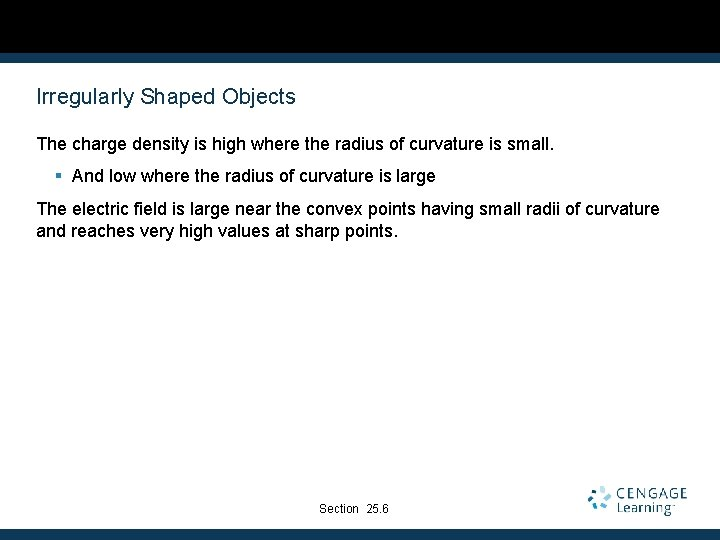Irregularly Shaped Objects The charge density is high where the radius of curvature is small. § And low where the radius of curvature is large The electric field is large near the convex points having small radii of curvature and reaches very high values at sharp points. Section 25. 6Cavity in a Conductor Assume an irregularly shaped cavity is inside a conductor. Assume no charges are inside the cavity. The electric field inside the conductor must be zero. Section 25. 6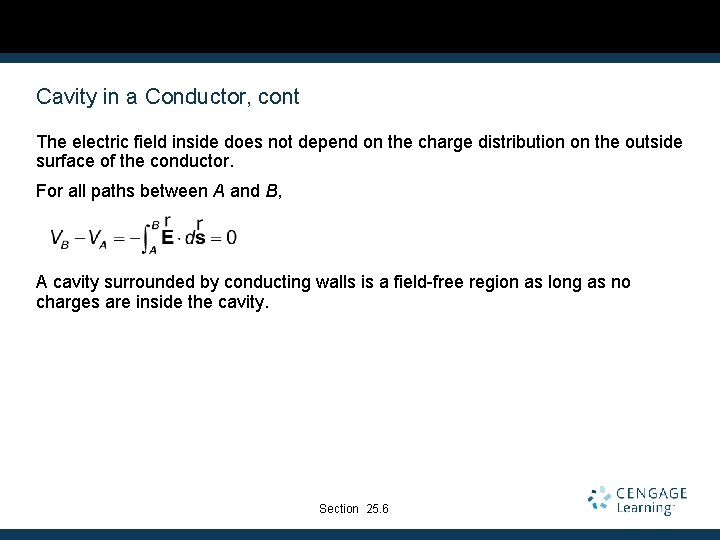Cavity in a Conductor, cont The electric field inside does not depend on the charge distribution on the outside surface of the conductor. For all paths between A and B, A cavity surrounded by conducting walls is a field-free region as long as no charges are inside the cavity. Section 25. 6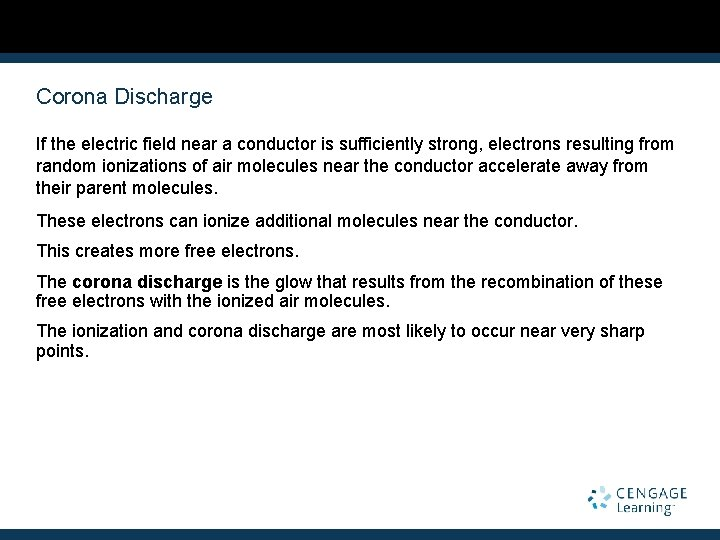Corona Discharge If the electric field near a conductor is sufficiently strong, electrons resulting from random ionizations of air molecules near the conductor accelerate away from their parent molecules. These electrons can ionize additional molecules near the conductor. This creates more free electrons. The corona discharge is the glow that results from the recombination of these free electrons with the ionized air molecules. The ionization and corona discharge are most likely to occur near very sharp points.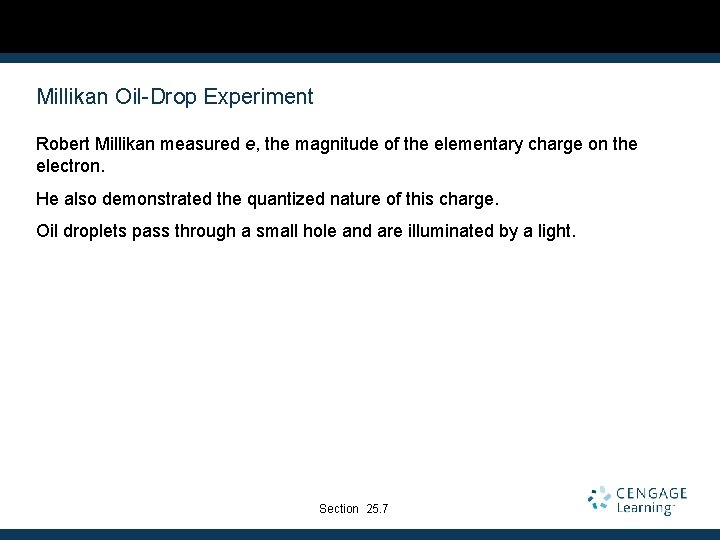Millikan Oil-Drop Experiment Robert Millikan measured e, the magnitude of the elementary charge on the electron. He also demonstrated the quantized nature of this charge. Oil droplets pass through a small hole and are illuminated by a light. Section 25. 7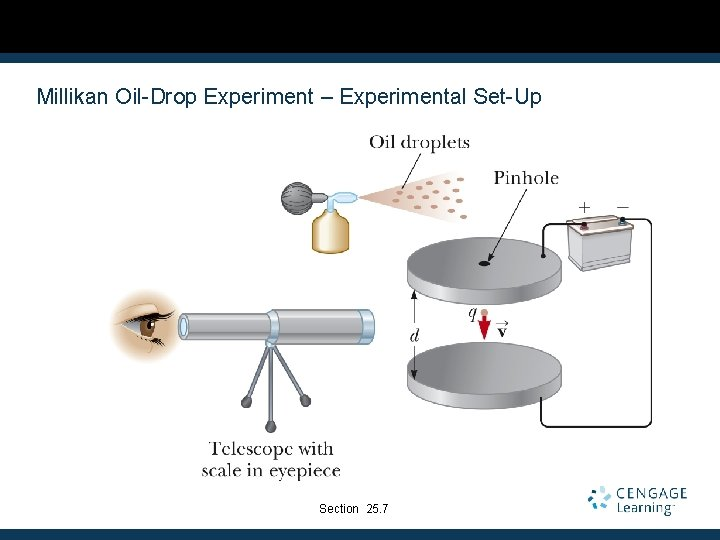Millikan Oil-Drop Experiment – Experimental Set-Up Section 25. 7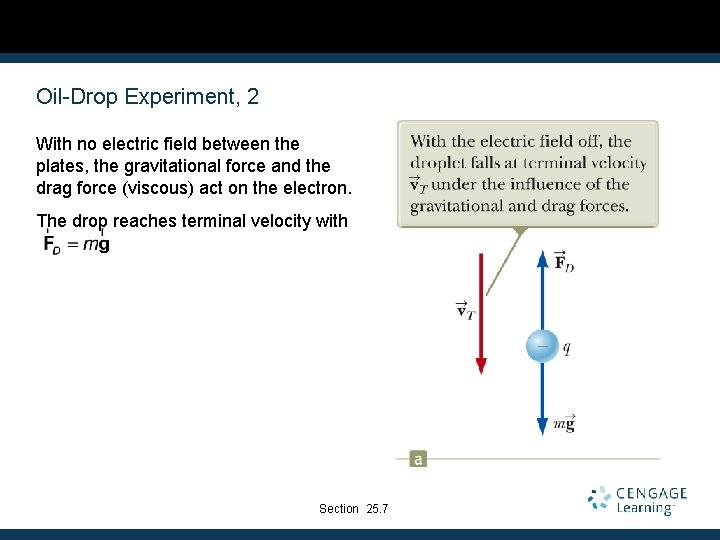Oil-Drop Experiment, 2 With no electric field between the plates, the gravitational force and the drag force (viscous) act on the electron. The drop reaches terminal velocity with Section 25. 7Oil-Drop Experiment, 3 An electric field is set up between the plates. § The upper plate has a higher potential. The drop reaches a new terminal velocity when the electrical force equals the sum of the drag force and gravity. Section 25. 7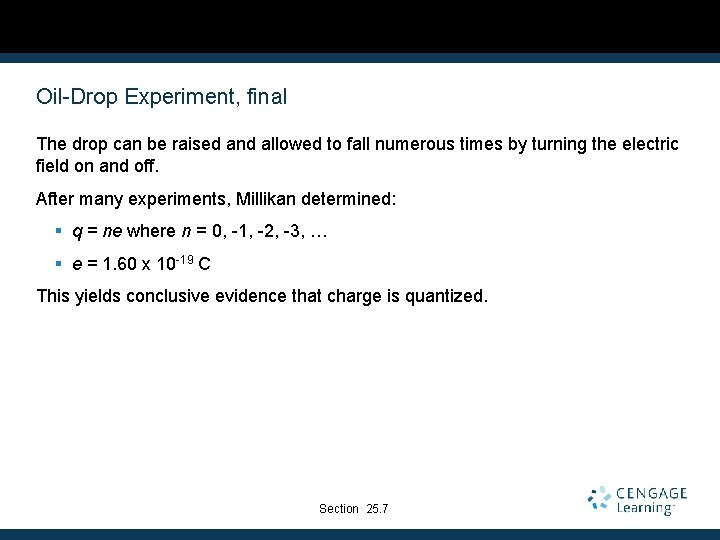Oil-Drop Experiment, final The drop can be raised and allowed to fall numerous times by turning the electric field on and off. After many experiments, Millikan determined: § q = ne where n = 0, -1, -2, -3, … § e = 1. 60 x 10 -19 C This yields conclusive evidence that charge is quantized. Section 25. 7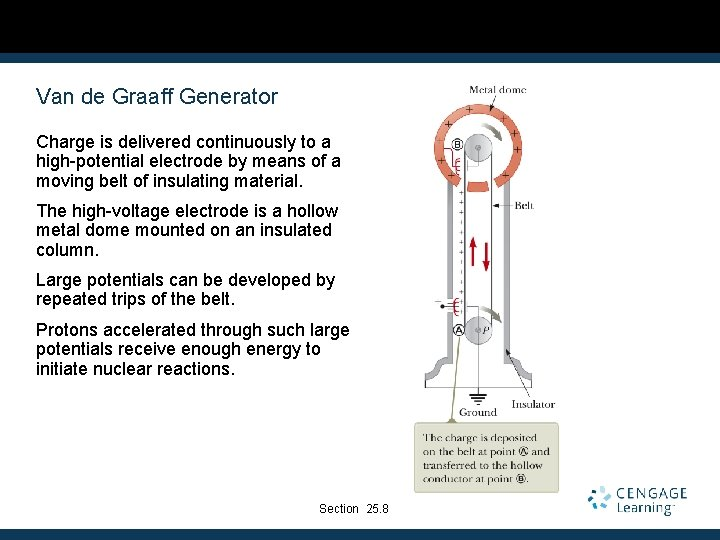Van de Graaff Generator Charge is delivered continuously to a high-potential electrode by means of a moving belt of insulating material. The high-voltage electrode is a hollow metal dome mounted on an insulated column. Large potentials can be developed by repeated trips of the belt. Protons accelerated through such large potentials receive enough energy to initiate nuclear reactions. Section 25. 8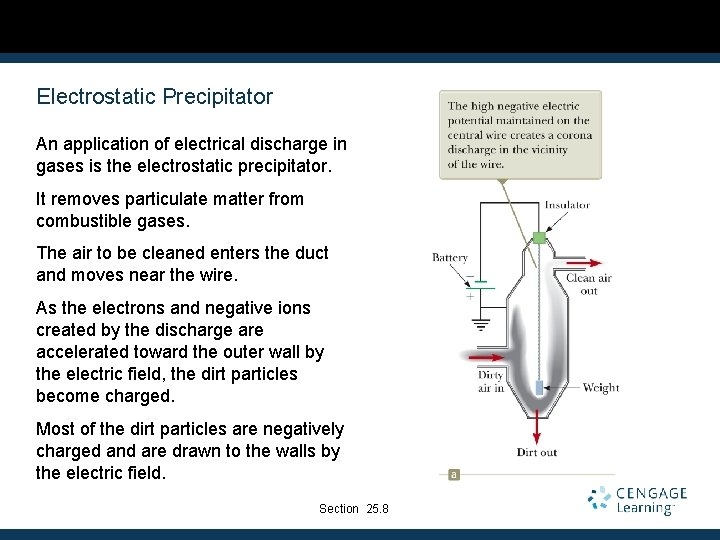Electrostatic Precipitator An application of electrical discharge in gases is the electrostatic precipitator. It removes particulate matter from combustible gases. The air to be cleaned enters the duct and moves near the wire. As the electrons and negative ions created by the discharge are accelerated toward the outer wall by the electric field, the dirt particles become charged. Most of the dirt particles are negatively charged and are drawn to the walls by the electric field. Section 25. 8